# Tangents at the Origin JEE Notes | EduRev

## JEE : Tangents at the Origin JEE Notes | EduRev

The document Tangents at the Origin JEE Notes | EduRev is a part of the JEE Course Mathematics (Maths) Class 12.
All you need of JEE at this link: JEE

B. Tangents at the Origin

If a curve passing through the origin be given by a rational integral algebraic equation, the equation of the tangent (or tangents) at the origin is obtained by equating to zero the terms of the lowest degree in the equation.

e.g., if the equation of a curve be x2 - 4y2 + x4 + 3x3y + 3x2 y2 + y4 = 0, the tangents at the origin are given by x2 - 4y2 = 0 or x + 2y and x - 2y = 0.

In the curve x2 + y2 + ax + by = 0, ax + by = 0, is the equation of the tangent at the origin; and in the curve (x2 + y2)= a2 (x2 - y2), x2 - y2 = 0 is the equation of a pair of tangents at the origin.

If the equation of a curve be x2 + y2 + x3 + 3 xy - y3 = 0, the tangents at the origin are given by x2 - y2 = 0 i.e. x + y = 0 and x - y = 0

C. Angle of intersection

Angle of intersection between two curves is defined as the angle between the two tangents drawn to the two curves at their point of intersection . If the angle between two curves is 90° then they are called ORTHOGONAL curves.

Ex.10 Find the angle between curves y2 = 4x and y = e-x/2

Sol.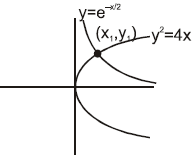Let the curves intersect at point (x1,y1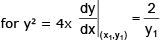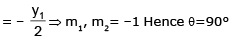Note : here that we have not actually found the intersection point but geometrically we can see that the curves intersect.

Ex.11 Show that the curves y = 2 sin2x and y = cos 2x intersect at π/6. What is their angle of intersection ?

Sol. Given curves are y = 2 sin2 x   ...(1)

and y = cos 2x             ...(2)

Solving (1) and (2), we get 2 sin2 x = cos 2x

⇒ 1 – cos 2x = cos 2x ⇒ cos2x = 1/2 ⇒ cos π/3 ⇒ 2x = ± π/3

x = ±π/6 are the points of intersection

From (1), dy/dx = 4 sin x cos x = 2 sin 2x = m1 (say)

From (2) dy/dx = -2 sin 2x = m2 (say)

If angle of intersection is θ, then tanθ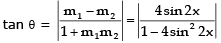∴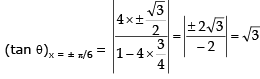∴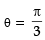Ex.12 Show that the angle between the tangents at any point P and the line joining P to the origin `O' is the same at all points of the curve ln (x2 + y2) = c tan-1 (y/x) where c is constant.

Sol. Let the point P(x, y) on the curve ln (x2 + y2) = c tan-1 (y/x) where c is constant.

Differentiating both sides w.r.t. x, we get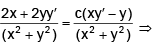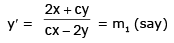Slope of OP  = y/x = m2 (say)

Let the angle between the tangents at P and OP be θ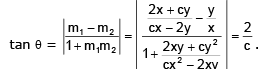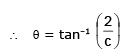which is independent of x and y..

Ex.13 Show tht the curves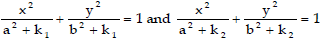intersect orthogonally.

Sol.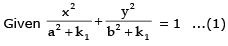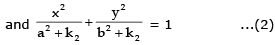Subtracting (2) from (1), we get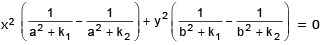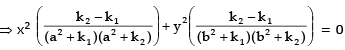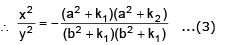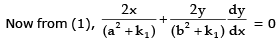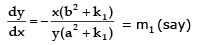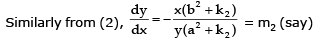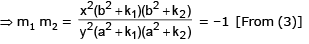Hence given curves intersect orthogonally.

Ex.14 Prove that the curves xy = 4 and x2 + y2 = 8 touch each other.

Sol.

Equation of the given curves are xy = 4 ....(i) and x2 + y= 8 ....(ii)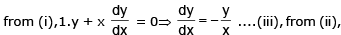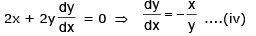Putting the value fo y from (i) i (ii), we get x2 + 16/x2 = 8 or or  x4 + 16 = 8x2

or  x– 8x2 + 16 = 0  or   (x2 – 4)2 = 0  or  x– 4 = 0  or  x2 = 4

from (i) ; when x = 2, y = 2 and when x = –2, y = -2

Hence points of intersection of the two curves are (2, 2) and (–2, –2).

Slope of the tangent to the curve (i) at point  (2, 2)  ⇒ m1 = -2/2 = -1 ...(from iii)

Slope of tangent to the curve (ii) at point (2, 2)   ⇒ m2 = -2/2 = -1....( from iv)

Since m3 = m4, hence the two curves touch each other at (-2, -2). Thus curves (i) and (ii) touch each other.

Slope of tangent to curve (i),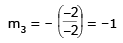Slope of tangent to curve (ii),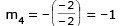Since m3 = m4, hence the two curves touch each other at (–2, –2). Thus curves (i) and (ii) touch each other.

Ex.15 The gradient of the common tangent to the two curves y = x2 - 5x + 6 & y = x2 + x + 1 is

(A) - 1/3

(B) - 2/3

(C) - 1

(D) - 3

Sol. y = ax + b on solving with both curves and putting D = 0 gives

a2 + 10a + 4b + 1 = 0 and a2 - 2a + 4b - 3 = 0 ⇒ a = - 1/3 & b = 5/9

⇒ 3x + 9y = 5 ; point of contact (7/3, - 2/9) & (- 2/3, 7/9)

D. Length of Tangent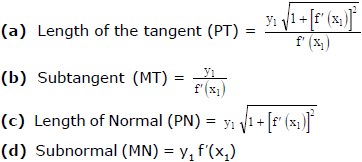Ex.16 What should be the value of n in the equation of curve y = a1 - n. xn, so that the sub-normal may be of constant length ?

Sol. Given curve is y = a1 - n . xn

Taking logarithm of both sides, we get, ln y = (1 - n) ln a + n ln x

Differentiating both sides w.r.tx, we get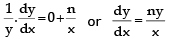...(1)

Lengths of sub-normal  = y dy/dx = y .ny/x ....{from 1}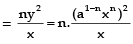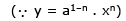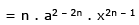Since lengths of sub-normal is to be constant, so x should not appear in its value i.e., 2n – 1 = 0. n =1/2

Ex.17 If the relation between sub-normal SN and sub-tangent ST at any point S on the curve

by2 = (x + a)3 is p(SN) = q(ST)2; then p/q is

(A) 8b/27

(B) b

(C) 1

(D) none of these

Sol.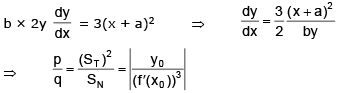Let a point by (x0, y0) lying on the curve by  = (x0 + a)3 ....(i)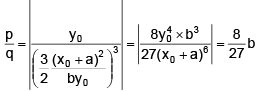(from equation (i))

Ex.18 For the curve y =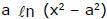show that sum of lengths of tangent & subtangent at any point is proportional to coordinates of point of tangency.

Sol.

Let point of tangency be (x1, y1)   ⇒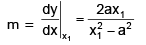tangent + subtangent  =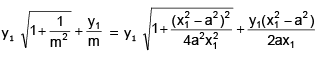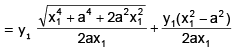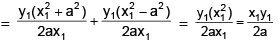Hence proved.

Ex.19 Show that the segment of the tangent to the curve y =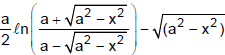contained between the y-axis and point of tangency has a constant length.

Sol.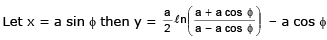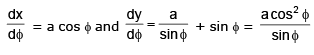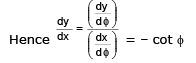Equation of tangent at ‘ø’   y – a ln cot ø/2 + a cos ø =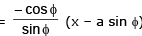⇒y sin ø – a sinø ln cot ø/2 + a sin ø cos ø - x cosø + a sin ø cos ø

⇒x cos ø+ y sin ø = a sin ø ln cot ø/2

Point on y-axis    P ≡ (0, a ln cot ø/2) and point of tangency

Q≡ (a sinø a ln cotø/2 – a cos ø)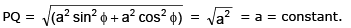E. Solving Equations

Ex.20 For what values of c does the equation ln x = cx2 have exactly one solution ?

Sol.

Let's start by graphing y = In x and y = cx2 for various values of c. We know that for c * 0, y = cx2 is a parabola that opens upward if c > 0 and downward if c < 0. Figure 1 shows the parabolas y = cx2 for several positive values of c. Most of them don't intersect y = In x at all and one intersect twice. We have the feeling that there must be a value of c (somewhere between 0.1 and 0.3) for which the curves intersect exactly once, as in Figure 2.

To find that particular value of c, we let 'a' be the x-coordinate of the single point of intersection. In other words, In a = ca2, so 'a' is the unique solution of the given equation. We see from Figure 2 that the curves just touch, so they have a common tangent line when x = a. That means the curves y = In x and y=cx2 have the same slope when x = a. Therefore 1/a = 2ca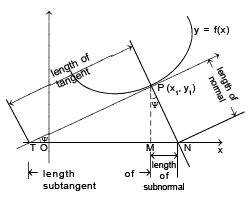Solving the equation In a = ca2 and 1/a = 2ca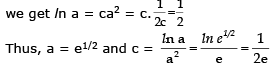For negative values of c we have the situation illustrated in Figure 3: All parabolas y = cx2 with negative values of c intersect y = In x exactly once. And let's not forget about   c = 0: The curve y = 0 x2 = 0 just he x-axis, which intersects y = In x exactly once.

To summarize, the required values of c are c=1/(2e) and c<0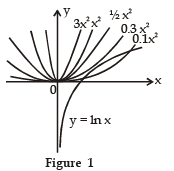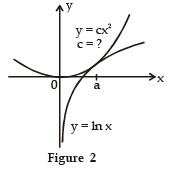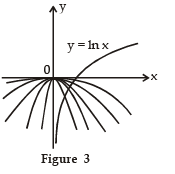Ex.21 The set of values of p for which the equation px= ln x possess a single root is

Sol.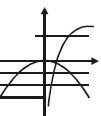for  p ≤ 0,  there is obvious one solution ;  for  p > 0  one root

⇒ the curves touch each .

2 px1 = 1/x1 ⇒   x12 = 1/2p ;

Also   px12 = ln x1 ⇒   p (1/2p) = ln x1 ⇒  x1 = e1/2

⇒   2 p = 1/e   ⇒   p = 1/2e .  Hence  p ∈ (- ∝, 0] U {1/2e}

F. Shortest distance

Shortest distance between two non-intersecting curves always along the common normal (wherever defined)

Ex.22 Find the shortest distance between the line y = x - 2 and the parabola y = x2 + 3x + 2.

Sol. Let P(x1, y1) be a point closest to the line y = x - 2 then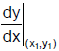= slope of line

⇒ 2x1 + 3 = 1 ⇒ x1 = - 1 ⇒ y1 = 0 Hence point (-1, 0) is the closest and its perpendicular distance from the

line y = x - 2 will give the shortest distance        ⇒ p =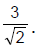Ex.23 Let P be a point on the curve C1: y =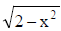and Q be a point on the curve C2: xy = 9, both P and Q lie in the first quadrant. If 'd' denotes the minimum value between P and Q, find the value of d2.

Sol. Note that C1 is a semicircle and C2 is a rectangular hyperbola.

PQ will be minimum if the normal at P on the semicircle is also a normal at Q on xy = 9

Let the normal at P be y = mx ....(1) (m > 0) solving it with xy = 9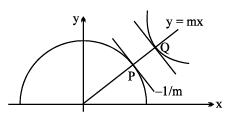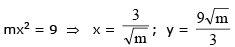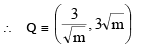differentiating xy = 9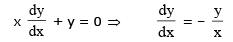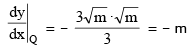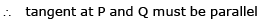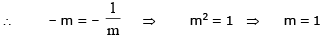∴ normal at P and Q is y = x

solving P(1, 1) and Q(3, 3)

(PQ)2 = d2 = 4 + 4 = 8

G. Rate Measurement

Ex.24 A ladder 10 ft long rests against a vertical wall. If the bottom of the ladder slides away from the wall at a rate of 1 ft/s, how fast is the top of the ladder sliding down the wall when the bottom of the ladder is 6 ft from the wall ?

Sol. We first draw a diagram and label it as in Figure 1. Let x feet be the distance from the bottom of the ladder to the wall and y feet the distance from the top of the ladder to the ground. Note that x and y are both function of t (time). We are given that dx/dt = 1 ft/s and we are asked to find dy/dt when x = 6 ft (see Figure 2). In this problem, the relationship between x and y is given by the Pythagorean Theorem : x2 + y2 = 100

Differentiating each side with respect to t using the Chain Rule, we have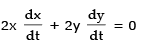and solving this equation for the desired rate, we obtain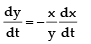When x = 6, the Pythagorean Theorem gives y = 8 and so, substituting these values and dx/dt = 1,

we have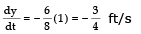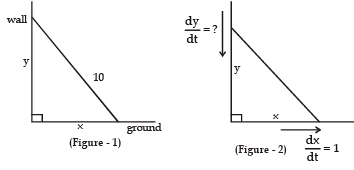The fact that dy/dt is negative means thatthe distance from the top of the ladder to the ground is decreasing at a rate of 3/4 ft/s In other words, the top of the ladder is sliding down the wall at a rate of  3/4 ft/s .

Ex.25 A water tank has the shape of an inverted circular cone with base radius 2 m and height 4 m. If water is being pumped into the tank at a rate of 2 m3/min, find the rate at which the water level is rising when the water is 3 m deep.

Sol.

We first sketch the cone and label it as in Figure. Let V, r, and h be the volume of the water, the radius of the surface, and the height at time t, where t is measured in minutes.

We are given that dV/dt = 2m3/min and we are asked to find dh/dt when h is 3 m. The quantities V and h are related by the equation V = 1/3pr2hBut it is the very useful to express V as a function of h alone.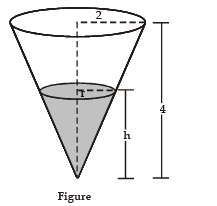In order to eliminate r, we use the similar triangles in Figure to write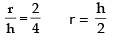and the expression for V becomes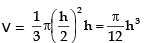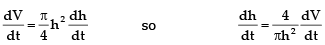Substituting h = 3 m and dV/dt = 2m3/min, we have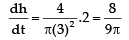The water level is rising at a rate of 8/(9p) ≈ 0.28 m/min.

Ex.26 A man walks along a straight path at the speed of 4 ft/s. A searchlight is located on the ground 20 ft from the path and is kept focused on the man. At what rate is the searchlight rotating when the man is 15 ft from the point on the path closest to the searchlight ?

Sol. We draw Figure and let x be the distance from the man to the point on the path closest to the searchlight. We let θ be the distance from the man to the point on the path closest to the searchlight and the perpendicular to the path.

We are given that dx/dt = 4 ft/s and are asked to find dq/dt when x = 15. The equation that relates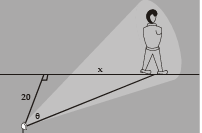Differentiating each side with respect to t, we get dx/dt = 20sec2 θ dθ/dt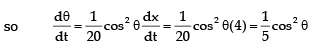when x = 15, the length of the beam is 25, so cosθ = 4/5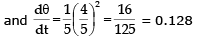The searchlight is rotating at a rate of 0.128 rad/s.

Ex.27 Two men A and B start with velocities v at the same time from the junction of two roads inclined at 45º to each other. If they travel by different roads, find the rate at which they are being separated.

Sol.

Let L and M be the positions of men A and B at any time t,

Let OL = x and LM = y. Then OM = x

given, dx/dt = v; to find dy/dt from ΔLOM,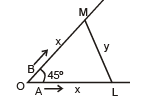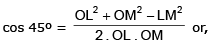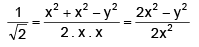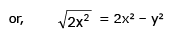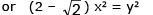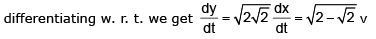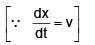∴ they are being separated from each other at the rate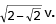Ex.28 A variable triangle ABC in the xy plane has its orthocentre at vertex 'B', a fixed vertex 'A' at the origin & the third vertex 'C' restricted to lie on the parabola y =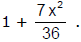The point B starts at the point (0, 1) at time t = 0 & moves upward along the y axis at a constant velocity of 2 cm/sec. How fast is the area of the triangle increasing when t = 7/2 sec ?

Sol.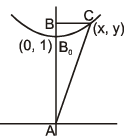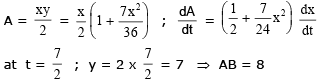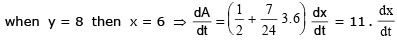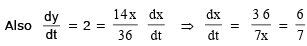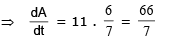Ex.29 Find the approximate value of (1.999)6.

Sol.

Let f(x) = x6. Now, f(x + δx) – f(x) = f'(x) . δx = 6x5 δx

We may write, 1.999 = 2 – 0.001

Taking x = 2 and δx = –0.001, we have f(1.999) – f(2) = 6(2)5 × – 0.001

⇒ f(1.999) = f(2) – 6 × 32 × 0.001 = 64 – 64 × 0.003 = 64 × 0.997 = 63.808 (approx).

Offer running on EduRev: Apply code STAYHOME200 to get INR 200 off on our premium plan EduRev Infinity!

## Mathematics (Maths) Class 12

209 videos|222 docs|124 tests

,

,

,

,

,

,

,

,

,

,

,

,

,

,

,

,

,

,

,

,

,

;